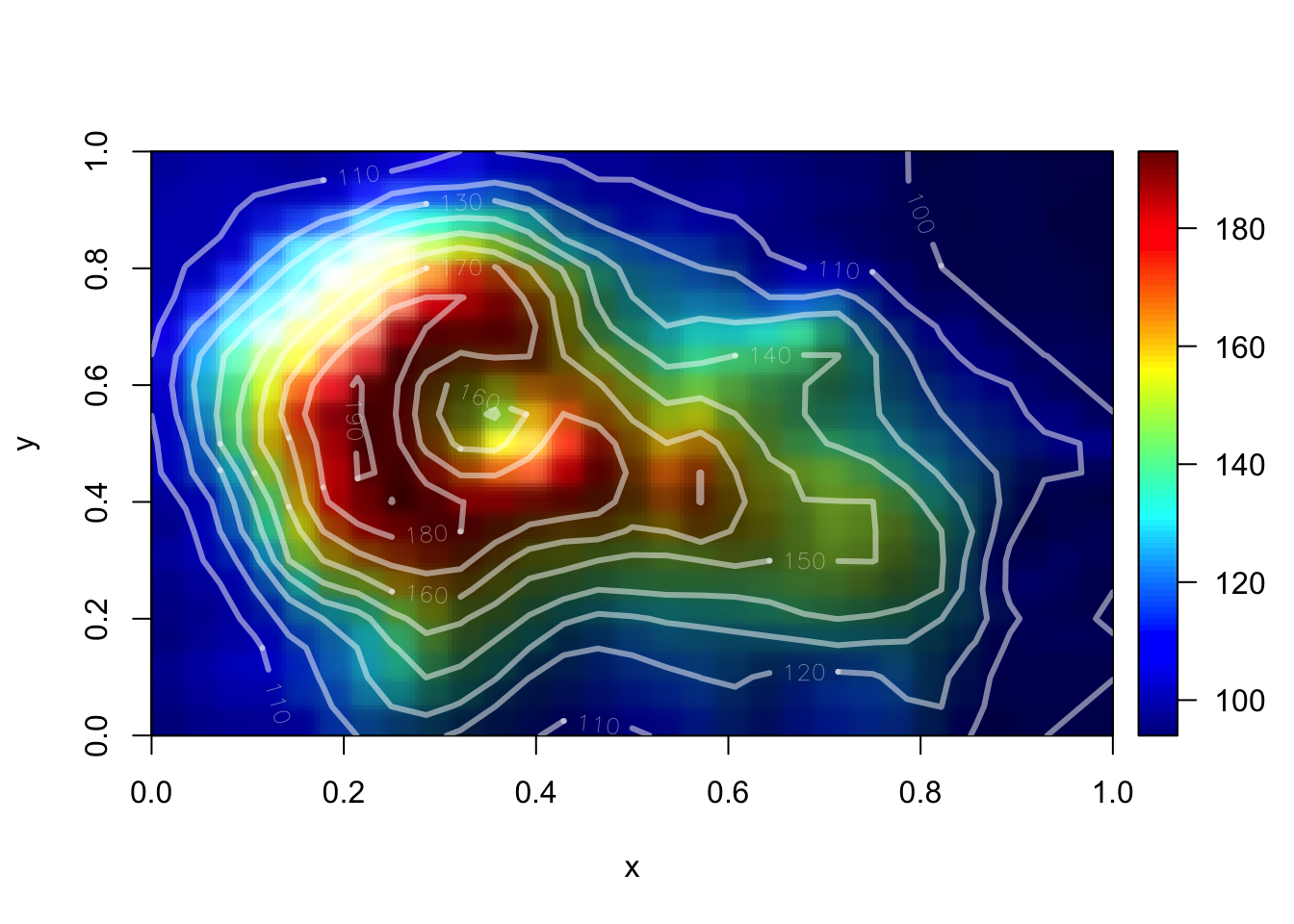# The Academia Theme in Hugo

This is really just a test to see if I can get this post to show up on the site. I thought that I would play with a bit of R code while I have the chance.

The code below is from: Fifty ways to draw a volcano using package plot3D by Karline Soetaert

``````# Preamble
library(plot3D)
# Reduce the resolution
Volcano <- volcano[seq(1, nrow(volcano), by = 3),
seq(1, ncol(volcano), by = 3)]``````
``````image2D(Volcano, lighting = TRUE, rasterImage = TRUE,
contour = list(col = "white", labcex = 0.8, lwd = 3, alpha = 0.5))``````### Related

comments powered by Disqus